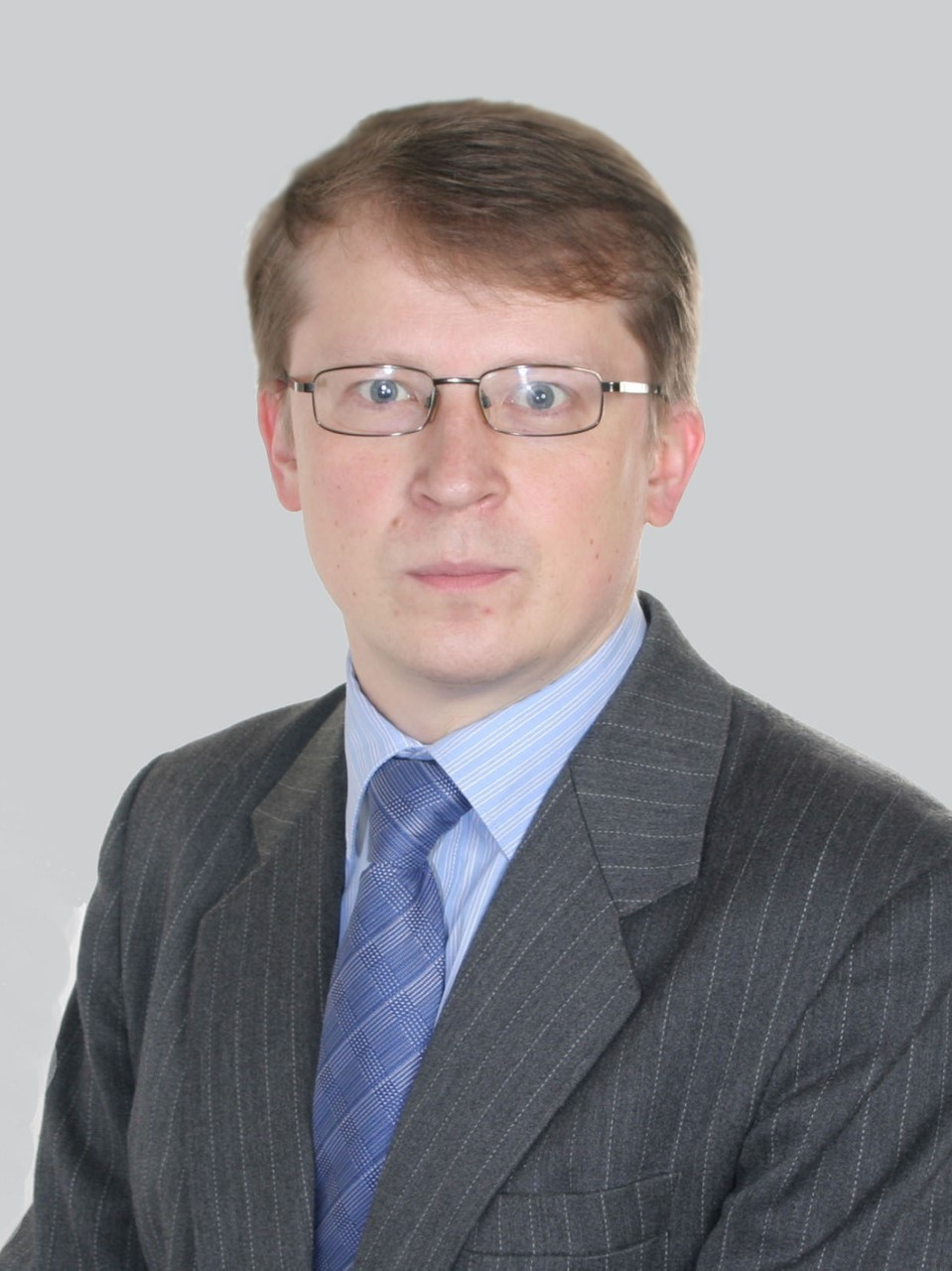Non-commutative elliptic theory for the actions of groups on manifolds
Non-commutative elliptic theory for the actions of groups on manifolds

Year 2021

The main goal of the project is to create a noncommutative elliptic theory for a new class of operators associated with the representation of a group by quantized canonical transformations on different varieties. We study the Fredholm property of the studied operators and index formulas are presented for them.

Issue

The project aims to explore a number of interconnected challenges of modern theory of elliptic operators on manifolds with the actions of groups. The ellipticity of such problems and their homotopic properties are investigated, index formulas are established.

Solution

Non-commutative elliptic theory is studied in the following geometric situations: (1) problems of relative elliptic theory (Sobolev problems), (2) problems on non-compact manifolds with group action. As part of this project, analytical, geometric and topological methods, both classical and those related to non-commutative geometry (A. Conn, A. Moscovici, R. Nest, B. Tsygan and others), as well as to coarse geometry (N. Higson, G.Yu., J. Roe) are developed.

Key publications on the project:

• A. Y. Savin, E. Schrohe, B. Y. Sternin Elliptic operators associated with groups of quantized canonical transformations. Successes of Mathematical Sciences. Vol. 73, 2018.
• A. Savin, E. Schrohe, B. Sternin Elliptic operators associated with groups of quantized canonical transformations. Bull. Sci. Math. 2018, 20p. (in print)
• P.A. Sipailo, Traces of quantized canonical transformations centered on a finite set of points. Differential Equations, 54, n. 4, 2018
• P.A. Sipailo, On traces of integral Fourier operators on subvarieties, Mathematical Notes, 2018 (in print)
• A. Y. Savin, B. Y. Sternin, “Elliptic problems with extensions-contractions on manifolds with boundary. C* - theory”, Differential Equations, 52 (2016), n. 10, 1383-1392.
• A. Y. Savin, B. Y. Sternin, Elliptic differential problems with extensions-contractions on manifolds with boundary. Differential Equations, 53 (2017), n. 5, 672-683
• A. Y. Savin, B. Y. Sternin, “Homotopy classification of elliptic problems, associated with actions of discrete groups on manifolds with boundary”, Ufa Mathematical Journal, vol. 8, No. 3, 2016, 126-134.
• A. Y. Savin, On homotopy classification of elliptic boundary value problems and problems with conjugation terms. Contemporary Mathematical Fundamental Directions. 2018, 8p.
• A. Y. Savin, B. Y. Sternin, “Elliptic G-operators on varieties with isolated singularities”, Contemporary Mathematics. Fundamental Directions, 59 (2016), 173-191.
• V. M. Manuilov. Pairs of operators with equal deviation from unitarity and their relative index. Matematicheskii Sbornik, 208 (2017), № 10, 113-125.
• A. Y. Savin, B. Y. Sternin, “On traces of operators associated with the actions of compact Lie groups”, Fundamental and Applied Mathematics. 2016. Vol. 21, n. 5.
• D.A. Loshchenova, On the traces of G-operators, focusing on submanifolds. Works of MIPT, Vol. 9, № 2(39), 2017, 85-96.
• N. R. Izvarina. On the symbol of nonlocal operators associated with a parabolic diffeomorphism. Eurasian Math. Journal, v.9, 2018, 11p.
Project goals
• The main goal of the project is - - to construct a noncommutative elliptic theory for a new class of operators, which we call G - operators associated with the representation of a group by quantized canonical transformations on different varieties. The operators under consideration are represented by three hypostases: 1) operators on closed varieties, 2) relative (noncommutative) elliptic theory, and 3) noncommutative elliptic theory on varieties with singularities. In all cases, the Fredholm character of the studied operators is studied and the index formulas for them are presented.Anton Savin

Doctor of Sciences, Professor, participant
Project results
The index problem for elliptic G-operators associated with the action of a discrete group of quantized canonical transformations is studied.
The relative index for balanced pairs of pseudo-differential operators whose symbols do not have to be elliptic and whose operators do not have to be Fredholm is studied.
Boundary value problems with nonlocal conditions are studied, in particular, problems associated with strains-compressions. Examples - problems for the perturbation of the Dirichlet problem for the Laplace operator in a ball by stretching and compression operators were analyzed. The problem of stable homotopic classification of elliptic operators with strains and compressions was also analyzed. This classification was obtained in the case where the compression acts identically on its attracting set.
Traces of G-operators associated with the action of a compact Lie group are studied for the case where the fixed points of the group action form a submanifold of arbitrary dimension embedded in a given submanifold. A complete description of such traces in terms of psedvo-differential operators with operator-valued symbols was obtained, the terms of their ellipticity were formulated, and the situation was reviwed when these traces are not Fredholm operators, but nevertheless they can be completed to Fredholm ones. Traces of quantized canonical transformations and, more generally, integral Fourier operators were also studied. The terms were formulated under which such traces are again integral Fourier operators.
The dependence of Fredholm solvability of parabolic diffeomorphisms of spheres on the smoothness index s in the scale of Sobolev spaces is studied. It is shown that the Fredholm solvability does not depend on s.
The conditions of ellipticity and index formula for the Sobolev problem with the boundary condition specified by the resolving operator for the wave equation on the submanifold have been obtained.
The finiteness theorem for elliptic complexes in relative elliptic theory was established. The results were applied to the relative de Rham complex and the de Rham relative theorem is established in a situation of smooth submanifolds.
Homotopic classification of G-operators for manifolds with cylindrical ends has been established.
Application area
• The results of the project have applications in the theory of inverse problems for hyperbolic equations, the theory of dynamical systems, as well as in noncommutative geometry.